## Transfer data from Android to PC using rsync

1. Install SimpleSSHD in your mobile

2. In order to start data transfers, you can use the rsync command which has the following syntax:

rsync [-switches] ORIGIN_PATH DESTINATION_PATH

To copy from phone to computer:

rsync --update --progress -e 'ssh -p 2222' -azv 192.168.1.112:/sdcard/DCIM/Camera /computer-path

To copy from computer to phone:

rsync --update --progress -e 'ssh -p 2222' -azv /computer-path 192.168.1.112:/sdcard/DCIM/Camera

The password will be autogenerated and shown in the mobile display once the rsync command is executed for every SSH connection.

## Introduction

Any two-terminal network whose internal circuitry is made up solely of resistors, current sources and voltage sources can be simplified to a voltage source ($$V_{TH}$$) with a resistor in series ($$R_{TH}$$). This is called Thevenin’s theorem.

In order the find the Thevenin’s equivalent circuit, it is necessary to follow two steps:

1. Find $$R_{th}$$ by turning off all internal sources and connecting an external current source.
2. Find $$V_{th}$$ by turning on all internal sources and leaving the external current source in open circuit.

When we turn off all internal supply sources we set $$v_{int} = 0$$, i.e., shorting the internal voltage supply sources, and we set $$i_{int} = 0$$, i.e., opening the internal current supply sources. Then, we apply an external current supply source ($$I_{ext}$$) and compute the correspondent voltage between the two terminals ($$V_{1}$$). The value of $$R_{TH}$$ will be:
$R_{th} = \frac{V_1}{I_{ext}}$

On the second step, we will turn on again all the internal sources and set the external current source as open circuit. The voltage seen at the two terminals of interest will be $$V_{th}$$

### ExampleWe will start the circuit analysis by setting all internal supply sources off. All the voltage sources will be shorted whereas the current sources will be left in open circuit.From here, now we are going to compute what is the voltage $$V_{1}$$.

Since there is no path to the negative terminal on $$R_1$$ and $$R_3$$ is shorted, they don’t contribute to the output voltage $$V_1$$.

Instead, $$V_1$$ can be computed as:
$V_1 = i_{ext} \cdot R_2$

Therefore, the Thevenin’s resistance is:
$R_{TH} = \frac{V_1}{i_{ext}} = \frac{i_{ext} \cdot R_2}{i_{ext}} = R_2$

Now we need to compute Thevenin’s voltage $$V_{TH}$$. For this we are going to turn on again all the internal sources, i.e., leaving the circuit as it originally was, and computing the voltage across its two terminals.If we apply the Kirchhoff’s current law (KCL) on every node:

$I_2 + i_3 = i_5$

$I_1 = i_3$

$i_5 = I_2 + i_4 + i_6$

From Equation 1, we find that

$i_1 + i_3 = \frac{V_{TH} – V_3}{R_2} \Rightarrow \left(i_1 + i_3\right) R_2 + V_3 = V_{TH}$

$V_{TH} = \left(0.5~mA + 1~{mA}\right) \cdot 10~{k}\Omega + 10~{V} = 25~V$

With this information, we can say that an equivalent circuit seen from these two terminals can be:## Current source and switched capacitors in parallel

A capacitor is an electronic device able to store electrical energy in an electrical field. Usually, the capacitor is defined in its most simple version as a device with two plates with area $$A$$, separated by air (or any other dielectric material) a distance $$d$$.By inductiveload – own drawing, done in Inkscape 0.44, Public Domain, Link

If a current source is forced through the capacitor, the electrons (charge) will be deposited in one of the plates, creating in turn a electrical field across them. There won’t be any effective charge transference from one plate to the other because the space between them is filled with a dielectric material (non conductive). However, the electrical field across it can force the repulsion or attraction of charge at the other side of the plate.

A capacitor is characterized by its capacitance. The capacitance is measured in Farads (F) and defines the ratio between the amount of charge needed to increase one volt at the terminals of the capacitor.

$C= \frac{Q}{V}$

Therefore, a capacitor with 1 F will need 1 Coulomb (1 C) of charge to set 1 V across its terminals. Remember, that 1 C represents the amount of energy transported by a constant current of 1 A in 1 second.

## Behavior of a capacitor connected to a DC current source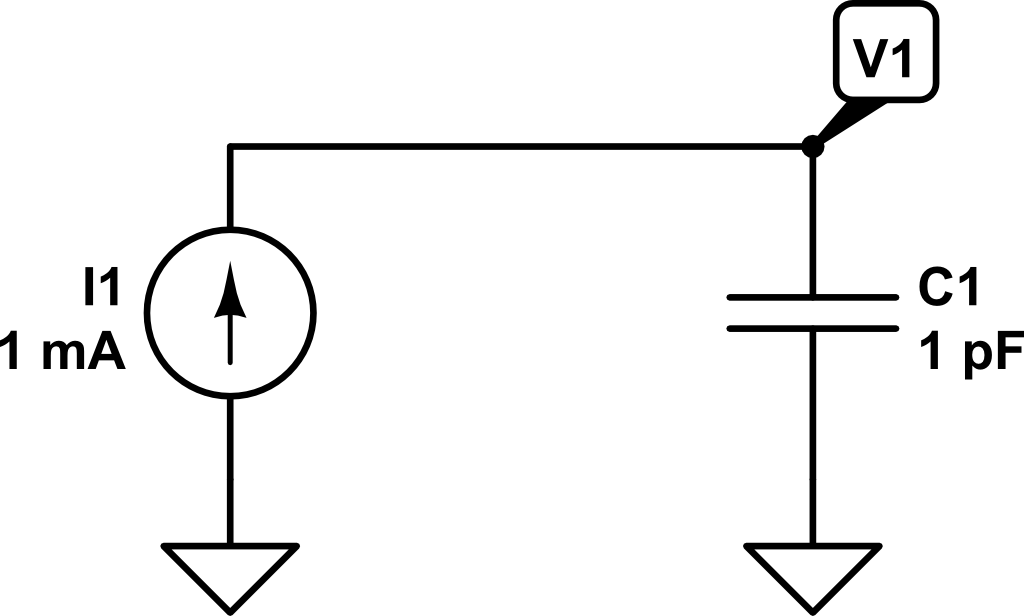Let’s see what happens when we connect a DC current source to a capacitor. Transforming a little bit the previous expression, we can obtain:

$C = \frac{Q}{V} \Rightarrow V = \frac{Q}{C}$

As $$Q = \int{i\left(t\right) dt}$$, we can get the voltage across the capacitor as a function of the time and the current:

$V\left(t\right) = \frac{1}{C} \int{i\left(t\right) dt}$

Therefore, if we inject a constant current of $$1~mA$$ for $$1~ns$$, the voltage $$V_1\left(t\right)$$ will be as follows: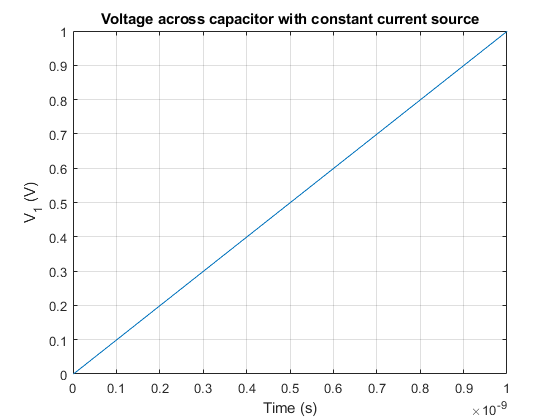In this example, what would be the charge stored in the capacitor at time $$t_1 = 1~ns$$?

$C = Q/V \Rightarrow Q = C \cdot V = 1~pF · 1V = 1\cdot10^{-12}~C$

Now, what would be the value of the voltage $$V_1$$ if at time $$t_1 = 1~ns$$ a second capacitor $$C_2$$ (discharged) of $$2~pF$$ of capacitance is connected?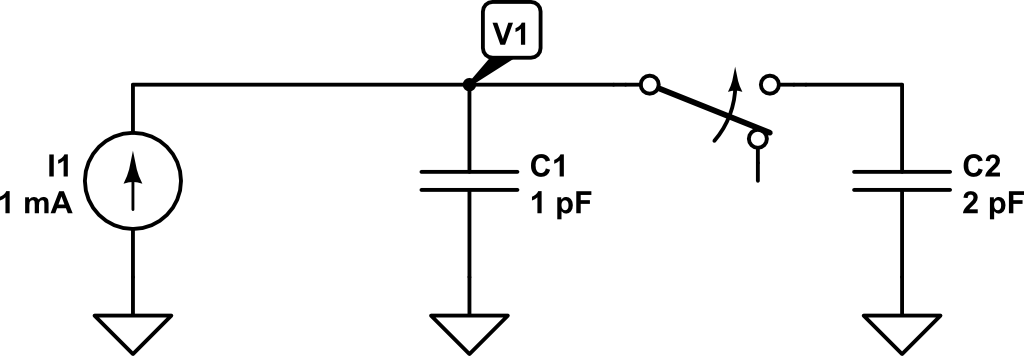At that very moment in which the second capacitor is connected in parallel, the charge in $$C_1$$ will be distributed between $$C_1$$ and $$C_2$$.

The effective capacitance now is $$3~pF$$ ($$1~pF + 2~pF$$). Remember that using the capacitance definition, we got:

$V = \frac{Q}{C_p} = \frac{Q}{C_1 + C_2} = \frac{1\cdot 10^{-12} C}{1\cdot10^{-12}~F + 2\cdot10^{-12}~F} = 0.333~V$

Therefore, as the capacitance now has increased and the charge remains the same (although shared between $$C_1$$ and $$C_2$$), according to the previous expression the voltage has to drop.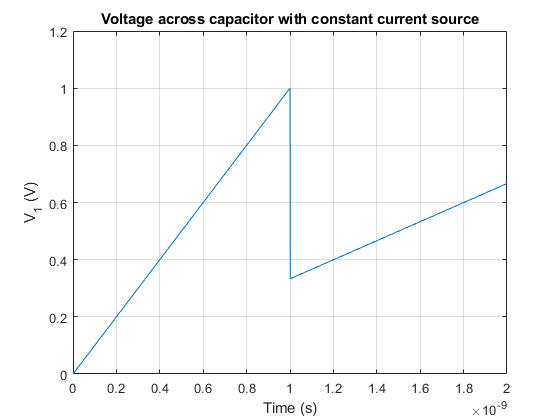If we would like to know the charge stored in every individual capacitor at time $$t_1 = 1~ns$$, we could compute it as:
$Q = C \cdot V$
$Q_1\left(t_1 = 1~ns\right) = C_1 \cdot V_1\left(t_1 = 1~ns\right) = 1~pF \cdot 0.333~V = 3.33\cdot10^{-13}~C$
$Q_2\left(t_1 = 1~ns\right) = C_2 \cdot V_1\left(t_1 = 1~ns\right) = 2~pF \cdot 0.333~V = 6.66\cdot10^{-13}~C$

The result is consistent with the previous calculation stating that the amount of charge at $$t_1=1~ns$$ was $$Q = 10^{-12}~C$$.

Finally, let’s see what happens if at time $$t_2 = 2~ns$$ the capacitor $$C_2$$ is again disconnected and only capacitor $$C_1$$ is connected to the current source.

At time $$t_2 = 2~ns$$ the voltage $$V_1$$ will have increased to:

$V\left(t_2 = 2~ns\right) = V_1\left(t_{1}^{+}\right) + \frac{1}{C_1 + C_2} \int_{t_1 = 1~ns}^{t_2 = 2~ns}{i\left(t\right) dt}$

$V\left(t_2 = 2~ns\right) = 0.333 V + \frac{1}{1~pF + 2~pF} \int_{t_1 = 1~ns}^{t_2 = 2~ns}{1~mA~dt} = 0.333~V +0.333~V = 0.666~V$

Now the charge will be distributed as follows:

$Q_1\left(t_2 = 2~ns\right) = C_1 \cdot V_1\left(t_1 = 2~ns\right) = 1~pF \cdot 0.666~V = 6.66\cdot10^{-13}~C$
$Q_2\left(t_2 = 2~ns\right) = C_2 \cdot V_1\left(t_1 = 2~ns\right) = 2~pF \cdot 0.666~V = 1.22\cdot10^{-12}~C$

If $$C_2$$ is disconnected, the charge stored in it will be lost and won’t be redistributed towards $$C_1$$. Therefore, the total amount of charge in the system at $$t_2 = 2~ns$$ will only be that on $$C_1$$.

Now, the voltage increment at the same rate as in the period $$0 < t < t_1 = 1~ns$$.

Therefore, if we plot the voltage from $$t=0~ns$$ to $$t_3 = 3~ns$$, we would get the following voltage profile for $$V_1$$: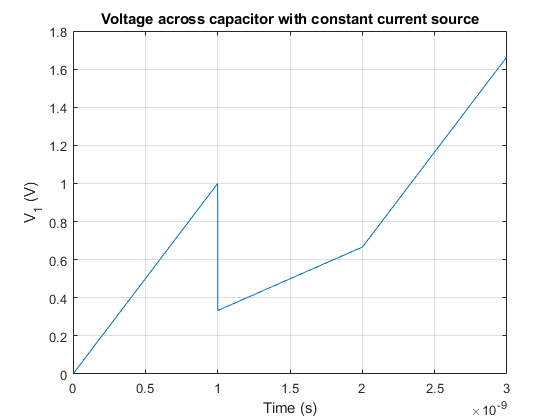## DFT as a change of basis

DFT can be thought as a simple change of basis. In the time domain, every sample of a signal represents its amplitude at that very instant.

$y\left[n\right] = \sin{\left( \frac{2\pi k }{N} n\right)}$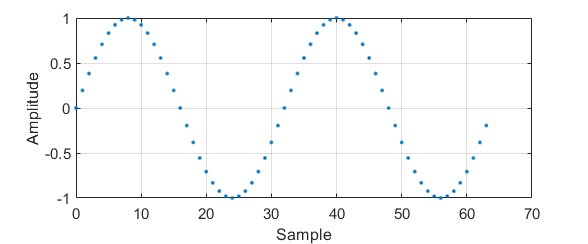$k = 2, N = 64$

Nevertheless, when applying a transformation such as the DFT, every sample means something different.

The DFT is computed as the scalar product between the signal and a orthogonal base. This base is as follows:

$\{\boldsymbol{w}^{(k)}\}_{\{k=0,1,…,N-1\}}$

$w_n = e^{j \frac{2\pi}{N}nk}$

If some of these base vectors are plotted with the imaginary part in the Y axe and the real part in the X axe:## DFT calculation

The DFT of a signal can be computed then, applying a change of basis over the desired signal.

$X\left[k\right] = \sum_{n=0}^{N-1} x\left[n\right] e^{-j\frac{2\pi}{N}nk}, \text{ } k = 0,1,…,N-1$

### DFT of a $$\delta\left[n\right]$$

$x\left[n\right] = \delta[n] = \left\{\begin{matrix} 1 & n = 0\\ 0 & otherwise \end{matrix}\right.$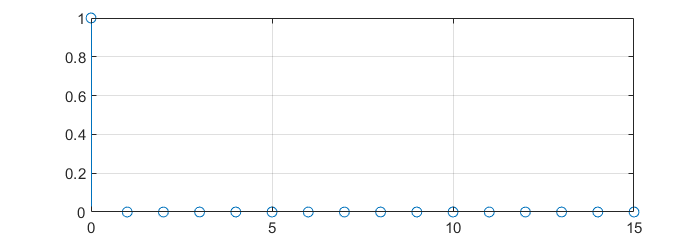$X\left[k\right] = \sum_{n=0}^{N-1}{\delta\left[n\right] e^{-j\frac{2\pi}{N}nk}}$

$X\left[k\right] = 1 \cdot e^{-j\frac{2\pi}{N}\cdot 0 \cdot k} = 1$

###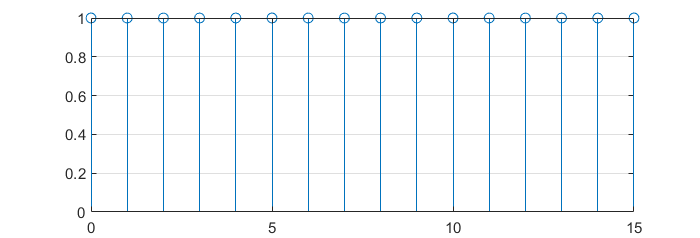DFT of a cosine

$x\left[n\right] = 4 \cos{\left(\frac{2\pi}{4}n\right)} = 4 \cos{\left(\frac{2\pi 4}{16}n\right)} = \frac{4}{2} \left[e^{ \frac{2\pi}{16} 4n} + e^{-j \frac{2\pi}{16} 4n} \right]$

[To be finished]

## Romancero bot: converting article text into voice with Amazon Polly and sending it to Telegram

The following Python script extracts the content of an article using Readibility (ported to Python), converts it to voice using the Amazon Polly service and finally sends the audio as a voice note to a given user in Telegram using Telethon (Telegram client for Python).

For running the script you will need to install the following Python packages:

pip install boto3
pip install awscli
pip install telethon

Also, you will need to create a AWS account. If you already have an AWS account, make sure that you have a user created in the IAM Management Console with the following permissions:

When creating this user, make sure you write down its ID access key and its secret access key. You will need them to configure the aws-cli client.

With this Amazon credentials, you can configure the AWS client by executing the following command:

aws configure

In this step, you will need to fulfill the details with the user credentials you wrote down when creating it.

Now, you will need to create a Telegram API ID. For this, you can go to the Telegram section «Create an Application«. After following the steps described in the official documentation, you will obtain an API ID (it’s a number) and a API hash (it’s a string).

With these steps already completed, you can place all the needed details in the script and run it.

import boto3
import textwrap
import requests
import re
from telethon import TelegramClient, events, sync
import time
import os

#--------------------------------------------------------------------
# Configuration
#--------------------------------------------------------------------

##### Article #####
# Define URL
url = "PLACE_THE_URL_HERE"

##### Telegram #####
# Telegram API credentials
api_id = PLACE_API_ID_HERE
api_hash = 'PLACE_API_HASH_HERE'
# Telegram user to send messages
telegram_user = "PLACE_TELEGRAM_USER_HERE"
# Create Telegram client
telegram_client = TelegramClient('session_name', api_id, api_hash)
telegram_client.start()

##### AWS configuration #####
# Get S3 session
session = boto3.Session(profile_name='default')
# Get polly client
polly = session.client('polly')
# Create a S3 client to retrieve the file content
s3 = session.client('s3')
# Define bucket name
bucket_name = 'PLACE_BUCKET_NAME_HERE'
#--------------------------------------------------------------------
# Get HTML from the article
#--------------------------------------------------------------------

# Get HTML
response = requests.get(url)

doc = Document(response.text)

# Get article body
html_text = doc.summary()

# Regular expression to identify HTML tags, e.g.:
html_tag_re = r"<\\?[^>]+>"

# Remove HTML tags from the article body
text_only = re.sub( html_tag_re, "", html_text,)

# Send message to user pointing to the URL that is going to be converted
telegram_client.send_message(telegram_user, "Converting: %s" % url)

#--------------------------------------------------------------------
# Convert text to voice
#--------------------------------------------------------------------

# Start Polly task to save in a Bucket
OutputS3BucketName=bucket_name,
Text=text_only,
VoiceId='Enrique')

# Monitor task status until it is completed
# Wait 2 seconds between server poll
time.sleep(2)
# Print the status of the task

#--------------------------------------------------------------------
# Retrieve file and send to Telegram user
#--------------------------------------------------------------------

# Regular expression to extract the key (file name) of the synthesized file
key_re = r'/([0-9A-Za-z-.]+)$' # Search the regular expression in the OutputUri regex_search = re.search(key_re, task['SynthesisTask']['OutputUri']) # Take only the first group of the re (key) file_key = regex_search.group(1) # Get file name to store in local title_sanitized = doc.short_title().replace('"', '') title_sanitized = title_sanitized.replace(':', '') file_name = "%s.mp3" % title_sanitized # Download file from the bucket and store it in a MP3 local file with open(file_name, 'wb') as data: s3.download_fileobj(bucket_name, file_key, data) # Delete remote bucket file s3.delete_object(Bucket=bucket_name,Key=file_key) # Delete local file if os.path.isfile(file_key): os.remove(file_key) # Send MP3 file as a voice note to the telegram user telegram_client.send_file(telegram_user, file_name, voice_note=True) # Send signature telegram_client.send_message(telegram_user, "Message sent from Romancero bot.") The user you specified will receive a message like this: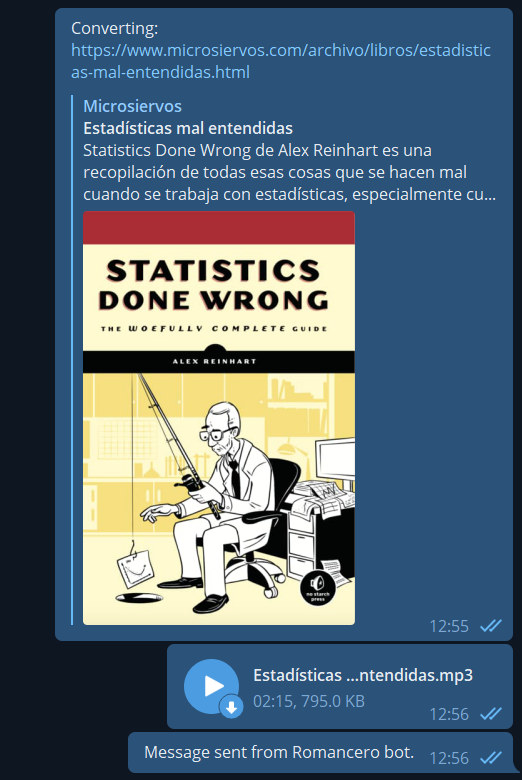## Parser in PHP using regular expressions You can use regular expressions in PHP with the function preg_match ( string$pattern , string $subject [, array &$matches [, int $flags = 0 [, int$offset = 0 ]]] ) . Only the first two paremeters are mandatory and they are the regex and the string where you want to search respectively.

In case of finding a result, preg_match() returns an array where the item at index 0 is the whole match. From 1 onwards they are placed the different groups of your regular expressions (in case there is any). If no match is found, preg_match() returns null.

One of the details that must be taken into account when using regular expressions on PHP is that they must be enclosed by forward slashes (/), e.g. $multiline_meaning_re = ‘/^([A-za-z ,»().\’;:]+)/’; . This regular expression matches any string with any set of letters, spaces, commas, double and single quotes, parenthesis points, colon and/or semicolon. As a complete example, the following snippet opens a file, parses it to look for English idioms and uploads all of them a MySQL database. <?php$servername = "";
$database = "";$username = "";
$password = "";$conn = mysqli_connect($servername,$username, $password,$database);

// Check connection
if (!$conn) { die("Connection failed: " . mysqli_connect_error()); } class Idiom { var$idiom = "";
var $meaning = ""; var$example = [];
function print(){
echo($this->idiom . "<br>" .$this->meaning . "<ul>");
print_r($this->example); if(count($this->example) == 0 )
echo("ERROR!!!!. There should be at least one example");
foreach ($this->example as$value) {
echo("<li>" . $value . "</li>"); } echo("</ul>"); } function upload($conn){
$example = "<ul class='list-group'>"; foreach ($this->example as $value) { if($value !== '')
$example =$example . "<li class='list-group-item'>" . $value . '</li>'; }$example = $example . "</ul>";$idiom = mysqli_real_escape_string($conn,$this->idiom);
$meaning = mysqli_real_escape_string($conn, $this->meaning);$example = mysqli_real_escape_string($conn,$example);

$query = 'INSERT INTO idioms (idiom, meaning, example) VALUES ("'.$idiom . '","'. $meaning . '","'.$example . '")';
mysqli_query($conn,$query);
}
}

$file = fopen("idioms.txt", "r") or die("Unable to open file!");$idiom_meaning_re     = '/^([A-za-z,. -\/()\']+):([A-za-z ,"().\';:\n]+)/';
$multiline_meaning_re = '/^([A-za-z ,"().\';:]+)/';$example_re           = '/^\|--([A-Za-z0-9 \',?.-;$\n"]+[^:])/';$new_idiom = 0;
$new_example = 0;$idiom = null;
$example = ""; // Output one line until end-of-file while(!feof($file)) {
$line = fgets($file);
//echo($line . "<br>"); preg_match($idiom_meaning_re, $line,$matches);

if($matches != null){ if($new_example === 1){
array_push($idiom->example,$example);
$new_example = 0; } if($idiom != null){
$idiom->print();$idiom->upload($conn); }$idiom = new Idiom;
$idiom->example = [];$example = "";
$new_idiom = 1;$idiom->idiom = trim($matches);$idiom->meaning = trim($matches); }else{ preg_match($example_re, $line,$matches);
if($matches != null){ if($example !== "")
array_push($idiom->example,$example);
$new_idiom = 0;$new_example = 1;
$example = trim($matches);
}else{
preg_match($multiline_meaning_re,$line, $matches); //var_dump($matches);
if($matches != null &&$new_idiom){
$idiom->meaning =$idiom->meaning . ' ' . trim($matches); //echo($idiom->meaning);
} elseif ($matches != null &&$new_example) {
$example =$example . ' ' . trim($matches); } else {$new_idiom   = 0;
$new_example = 0; } } } } echo("Closing file"); fclose($file);

mysqli_close($conn); ?>   You can find further information about the preg_match() in the PHP official documentation. ## Introduction to D3 (Data-Driven Document) One of the first questions you may ask yourself when getting introduced in D3 is: why are we using selectAll(‘html-tag-name’) method if there is no item to select of that type? First, let’s see an example of the situation we are talking about: <body> <script> const dataset = [12, 31, 22, 17, 25, 18, 29, 14, 9]; // Add your code below this line d3.select('body') .selectAll('h2') .data(dataset) .enter() .append('h2') .text('New Title') // Add your code above this line </script> </body> In the previous example, the only existing HTML tag is <body> . We select body (d3.select(‘body’) ) and then we perform the .selectAll(‘h2’) . On its own, it makes no sense as the method won’t return any value since no <h2> tag exists. Nevertheless, it will make sense if we keep looking at the following code. After the select .selectAll(‘h2’) we attach the existing dataset to the selected items (.data(dataset) ). Then, we use the enter() method, which gives meaning to the previous .selectAll(‘h2’) . When using enter() , D3 looks for the number of selected items to bind them with the data. In case of having not enough items in the selection, the enter() method will create them. Therefore, as .selectAll(‘h2’) was empty and the dataset variable contains 9 elements, it will iterate the code 9 times. In case of having already created some <h2> elements, it will simply fulfill the HTML code the necessary iteration to cover all the dataset elements. Remember that who does this iteration is the data() method. It parses the data set, and any method that’s chained after data() is run once for each item in the data set. You can find more information in the official documentation at the D3js.org website. ### Scales In D3 there exists the Scale function to change the value of the data set so that it can fit in the screen. Two important methods are range() and domain(). The domain method covers the set of input values whereas the range function convers the set of output values. Let’s see an example: const scale = d3.scaleLinear(); scale.domain([50, 480]); scale.range([10, 500]); scale(50) // Returns 10 scale(480) // Returns 500 scale(325) // Returns 323.37 scale(750) // Returns 807.67 From freeCodeCamp: The domain() method passes information to the scale about the raw data values for the plot. The range() method gives it information about the actual space on the web page for the visualization. ## Send audio from mobile phone (Android/iOS) to Raspberry Platform used: Raspberry Pi 3 B+ Bluetooth module: built-in First, update firmware to make sure you have latest version: sudo rpi-update Now, install all the needed pulse-audio packages:  sudo apt install pulseaudio-*. Configure the bluetooth in the Raspberry: pi@raspberrypi:~$ bluetoothctl
[NEW] Controller XX:XX:XX:XX:XX:XX raspberrypi [default]
[bluetooth]# power on
Changing power on succeeded
[bluetooth]# agent NoInputNoOutput
Agent registered
[bluetooth]# default-agent
Default agent request successful
[bluetooth]# discoverable on
Changing discoverable on succeeded
[CHG] Controller B8:27:EB:DB:58:46 Discoverable: yes
[bluetooth]# pairable on
Changing pairable on succeeded


In your mobile phone, search for the raspberry bluetooth signal and pair to it:

[Noname]# connect XX:XX:XX:XX:XX:XX
Attempting to connect to XX:XX:XX:XX:XX:XX
[CHG] Device XX:XX:XX:XX:XX:XX ServicesResolved: yes
Connection successful
[Noname]# trust XX:XX:XX:XX:XX:XX
Changing XX:XX:XX:XX:XX:XX trust succeeded
[CHG] Controller XX:XX:XX:XX:XX:XX Discoverable: no
[Noname]# exit

Now, you can try to play some audio in your mobile and it should be reproduced in the the Raspberry.
In my case, audio was driven out to the HDMI. In case you want to switch it through the Jack 3.5 mm output you can run:

sudo raspi-config

Then, select Advanced Options > Audio > Force 3.5 mm (‘headphone’) jack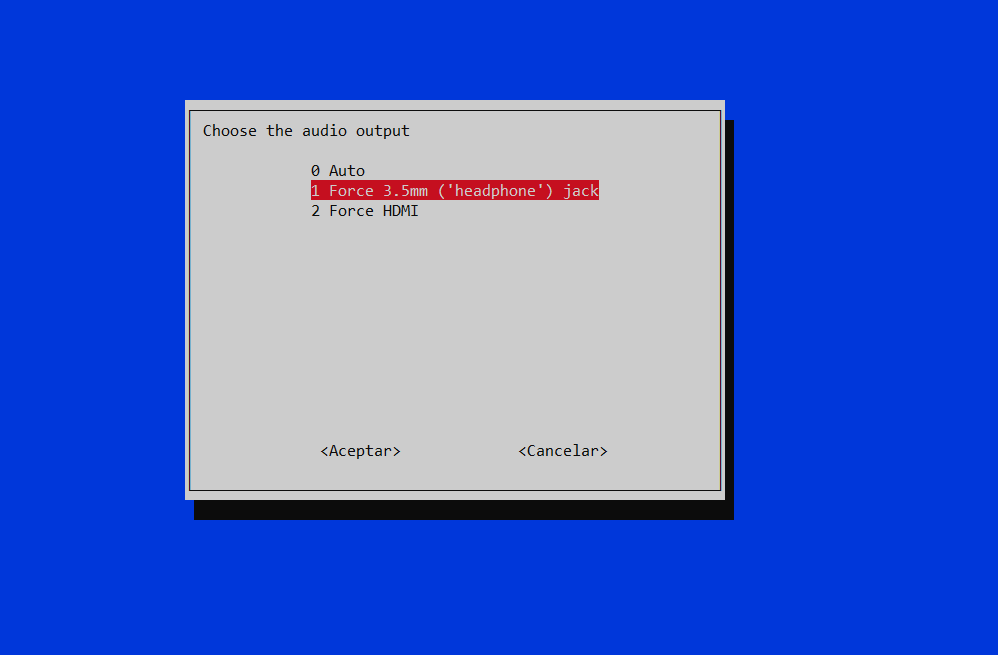With this all set, you can stream any audio such as the built-in music player, Spotify or YouTube to the Raspberry and from it to the connected speakers or HDMI display.

Finally, as the device has been stored as a trusted device, every time the Raspberry is booted, you won’t need to repeat this process. It will be so easy as connecting your mobile phone to the available raspberry bluetooth signal.

If sound sounds distorted, try restarting pulseaudio with:

pulseaudio -k

## Handling with several devices

If you pair several devices, only first connected device will be able to stream audio. If I connect my computer to the raspberry and then I try to connect my mobile phone (both previously paired and trusted), phone connection will fail. First, I’ll need to disconenct my computer and only then I’ll be able to successfully connect to the raspberry with my mobile phone.

## Web scraper with Scrapy

#!/usr/bin/env python
# -*- coding: utf-8 -*-

import scrapy
import re

title_regex       = r'Letra de\s+([a-zA-Z0-9áéíóúñü_,!¡¿?"() ]+)\s-'
empty_lines_regex = r"^\s+$" tabs_regex = r"^[\n\t]+" class ConchaPiquerSpider(scrapy.Spider): name = 'conchitabot' allowed_domain = ['http://www.coveralia.com'] start_urls = ['http://www.coveralia.com/letras-de/concha-piquer.php'] custom_settings = { 'FEED_EXPORT_ENCODING': 'utf-8', } BASE_URL = 'http://www.coveralia.com' def parse(self, response): lyric_links = response.css(".lista_uno li a::attr(href)").extract() for link in lyric_links: absolute_url = self.BASE_URL + link yield scrapy.Request(absolute_url, callback=self.parse_lyric) lyric_names_raw = response.css(".lista_uno li a::text").extract() def parse_lyric(self,response): raw_titles = response.css("h1").extract() for raw_title in raw_titles: match = re.search(title_regex, raw_title.encode("utf-8")) if match: title = match.group(1) raw_text = response.css("#HOTWordsTxt::text").extract() encoded_text = [] single_string = "" for item_text in raw_text: single_string = single_string + item_text lyric = self.clean_lyric(single_string) text_file = open("./letras/" + title + ".txt", "w") text_file.write(lyric) text_file.close() def clean_lyric(self,dirty_str): encoded = dirty_str.encode("utf-8") no_spaces = re.sub(r"^\s+", '', encoded) no_tabs = re.sub(r"[\n\t]+", '', no_spaces) return no_tabs #!/usr/bin/env python # -*- coding: utf-8 -*- import os import re def get_sorted_files(Directory): filenamelist = [] for root, dirs, files in os.walk(Directory): for name in files: fullname = os.path.join(root, name) filenamelist.append(fullname) return sorted(filenamelist) text = "<head><meta charset='utf-8'>" folder = "./letras/" files = get_sorted_files(folder) for filename in files: filebase = re.sub(folder, "", filename) filebase = re.sub("\..*$", "", filebase)
with open(filename,'r') as f:
text = text + "<h1>" + filebase + "</h1><pre>" + f.read() + "</pre>"

unified = open("unified.html", "w")
unified.write(text)
unified.close()

## Basis in a vector space

A vector space basis is the skeleton from which a vector space is built. It allows to decompose any signal into a linear combination of simple building blocks, namely, the basis vectors. The Fourier Transform is just a change of basis.

A vector basis is the linear combination of a set of vector that can write any vector of the space.

$w^{(k)} \leftarrow \text{basis}$

The canonical basis in $$\mathbb{R}^2$$ are:

$$e^{(0)} = [1, 0]^T \ \ e^{(1) } = [0,1]^T$$

Nevertheless, there are more basis for $$\mathbb{R}^2$$:

$$e^{(0)} = [1, 0]^T \ \ e^{(1) } = [1,1]^T$$

This former basis is not linearly independent as information of $$e^{(0)}$$ is inside $$e^{(1)}$$.

## Formal definition

H is a vector space.

W is a set of vectors from H such that $$W = \left\{ w^{(k)} \right\}$$

W is a basis of H if:

1. We can write $$\forall x \in H$$: $$x = \sum_{k=0}^{K-1} \alpha_k w^{(k)}, \ \ \alpha_k \in \mathbb{C}$$
2. $$\alpha_k$$ are unique, namely, there is linear independence in the basis, as a given point can only be expressed in a unique combination of the basis.

Orthogonal basis are those which inner product returns 0:

$$\left \langle w^{(k)}, w^{(n)} \right \rangle = 0, \ \ \text{for } k \neq n$$

In addition, if the self inner product of every basis element return 1, the basis are orthonormal.

## How to change the basis?

An element in the vector space can be represented with a new basis computing the projection of the current basis in the new basis. If $$x$$ is a vector element and is represented with the vector basis $$w^{(K)}$$ with the coefficients $$a_k$$, it can also be represented as a linear combination of the basis $$v^{(k)}$$ with the coefficients $$\beta_k$$. In a mathematical notation:

$x = \sum_{k=0}^{K-1} \alpha_k w^{(k)} = \sum_{k=0}^{K-1} \beta_k v^{(k)}$

If $$\left\{ v^{(k)} \right\}$$ is orthonormal, the new coefficients $$\beta_k$$ can be computed as a linear combination of the previous coefficients and the projection of the new basis over the original one:

$\beta_h = \left \langle v^{(h)}, x \right \rangle = \left \langle v^{(h)}, \sum_{k=0}^{K-1} \alpha_k w^{(k)} \right \rangle = \sum_{k=0}^{K-1} \alpha_k \left\langle v^{(h)}, w^{(k)} \right \rangle$

This operation can also be represented in a matrix form as follows:

$\beta_h = \begin{bmatrix} c_{00} & c_{01} & \cdots & c_{0\left(K-1 \right )}\\ & & \vdots & \\ c_{\left(K-1 \right )0} & c_{\left(K-1 \right )01} & \cdots & c_{\left(K-1 \right )\left(K-1 \right )} \end{bmatrix}\begin{bmatrix} \alpha_0 \\ \vdots \\ \alpha_{K-1} \end{bmatrix}$

This operation is widely used in algebra. A well-known example of a change of basis could be the Discrete Fourier Transform (DFT).